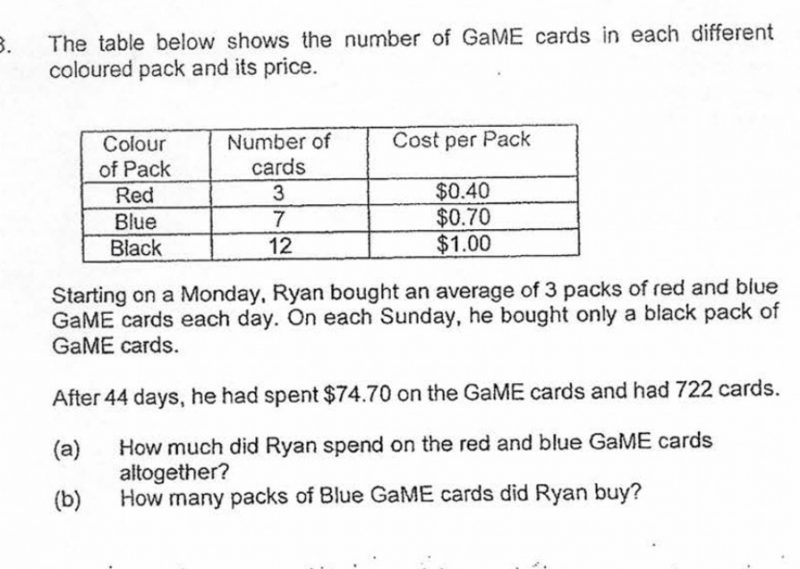# Questionhi, can help with this qn? tq!

Source: Rosyth

a)

44 Days / 7 = 6 R2  ***(6 full weeks and extra 2 days, Since it start on Monday)

6 Weeks = 6 Sundays

Sunday only buy Black = 6 * \$1

= \$6

Total Spend on Red and Blue = \$74.70 – \$6

= \$68.70

b)

Days buying Blue and Red = 44 – 6

= 38 Days

3 pack a day = 38 * 3

= 114

If all is Red Pack = 114 * \$0.40

= \$45.60

Difference from Total of Blue and Red = \$68.70 – \$45.60

= \$23.10

Price difference of Blue and Red Pack = \$0.70 – \$0.40

= \$0.30

Total Blue Pack = 23.10 / 0.30

= 77 Blue Pack